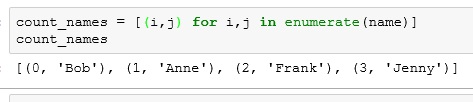# Python: Intro to Graphs

Visualizations are big part of analytics. You will need to produce visually engaging graphics for presentations, reports, and dashboards. You will also make graphs for your own use in data discovery and analysis. As bonus, unlike data cleaning, data viz can be pretty fun.

## Matplotlab and Pyplot

Matplotlab is a module you can import into Python that will help you to build some basic graphs and charts. Pyplot is part of Matplotlab and the part we will be using in the following example.

**If you are using the Anaconda Python distribution, Matplotlab is already installed. If not, you may need to download it from another source.

## Line Graph

Syntax

• %matplotlib inline – this code allows you to view your graphs inside jupyter notebooks
• from matplotlib import pyplot as plt – here we import pyplot from matplotlib into our program (note, we only want pyplot not all the functions in matplotlib).Adding “as plt” gives us a shorter alias to work with
• age and height lines – fill our lists with age and height information for an individual
• plt.plot(age, height, color = ‘blue’) – here we tell Python to plot age against height and to color our line blue
• plt.show() – prints out our graph## Bar Chart

For this example, we will make a bar charting showing ages of 4 people.

Syntax

• You should understand the first few lines from the first example
• count_names = [i for i,_ in enumerate(name)]  – since the name list is a list of strings, we cannot really graph that onto a chart. We need a way to convert these strings into numbers.

Wait? What does for i,_ mean? Let’s jump to the next code sampleWhile you don’t see it when making the list, a Python list is technically a list of tuples (index number, element). So if instead of i,_ we asked for both elements in the tuple, (i,j) we would get the following.So by iterating by for i,_ we only return the first element in the tuple (the index)

** notice we are using a list comprehension. If you are unfamiliar with list comprehensions, check out my earlier post: Python: List Comprehension

Let’s clean up our bar chart a little now.

• plt.ylabel(‘Age’) – label the y-axis Age
• plt.title(‘Age of People’) – give the graph a title
• plt.xticks([i+0.5 for i,_ in enumerate(name)], name) – this label function is using a list comprehension to first chose the position on the X-axis, and name provides the person’s name for the label.If you enjoyed this lesson, click LIKE below, or even better, leave me a COMMENT

1.BenKotey
1.Ben Larson